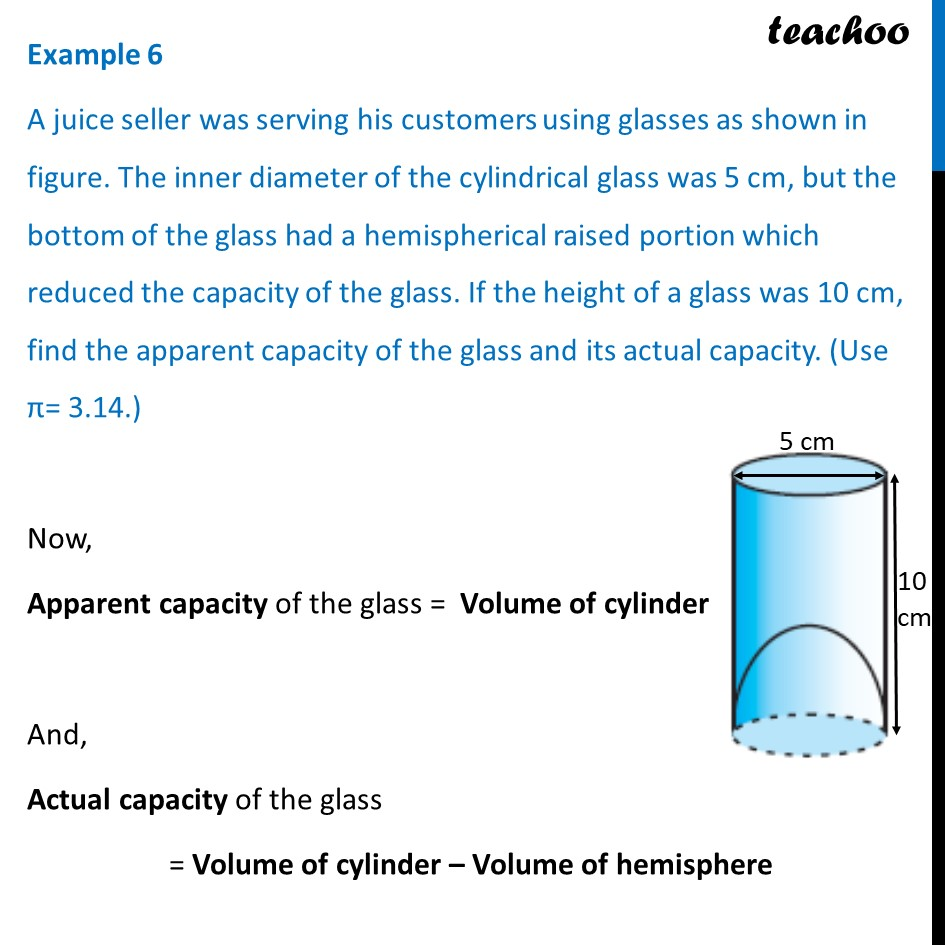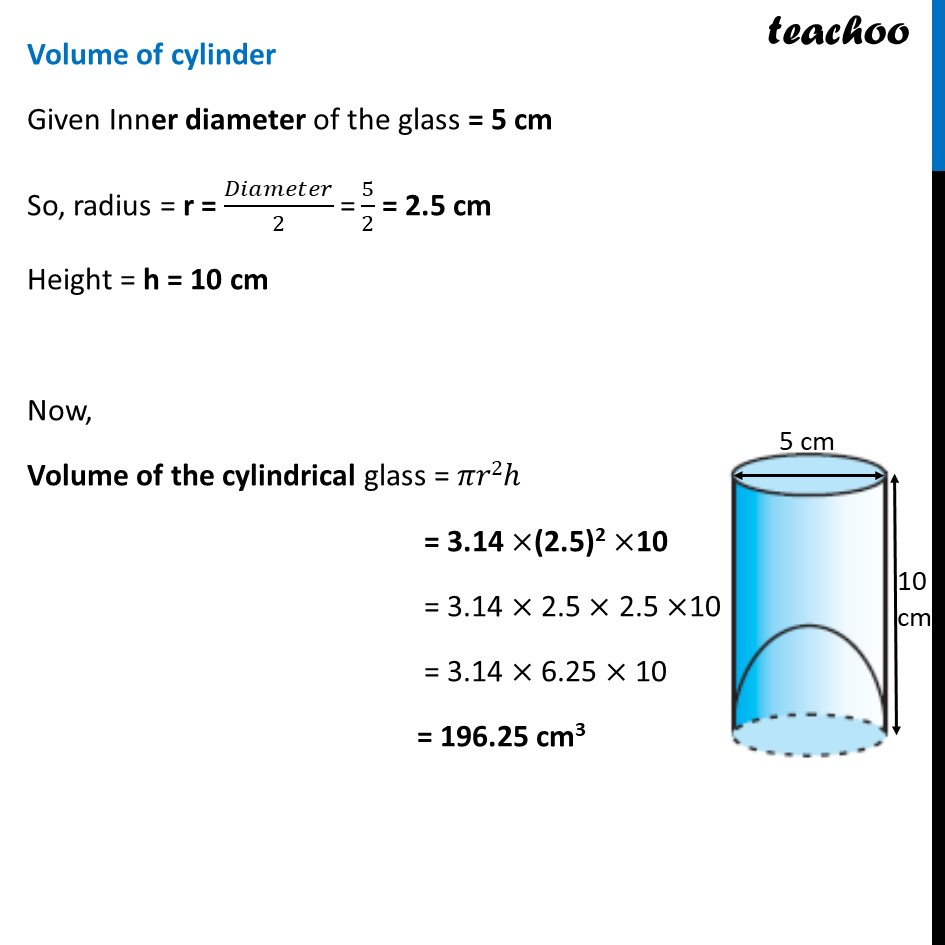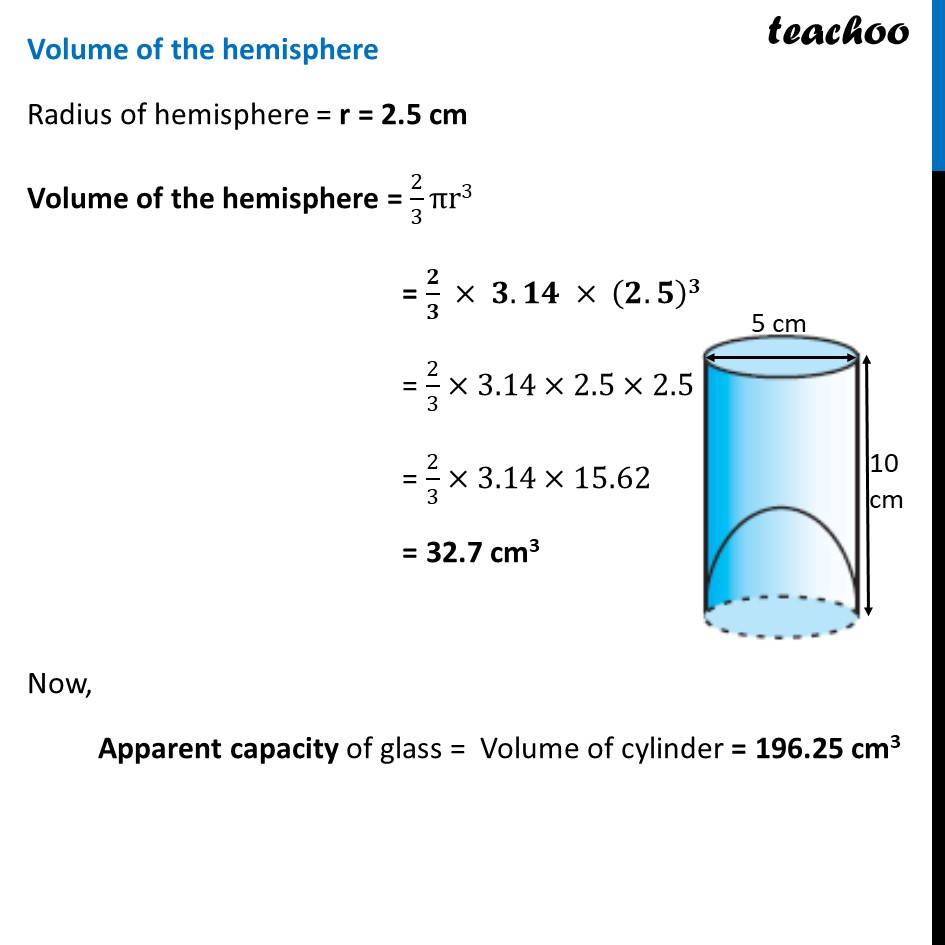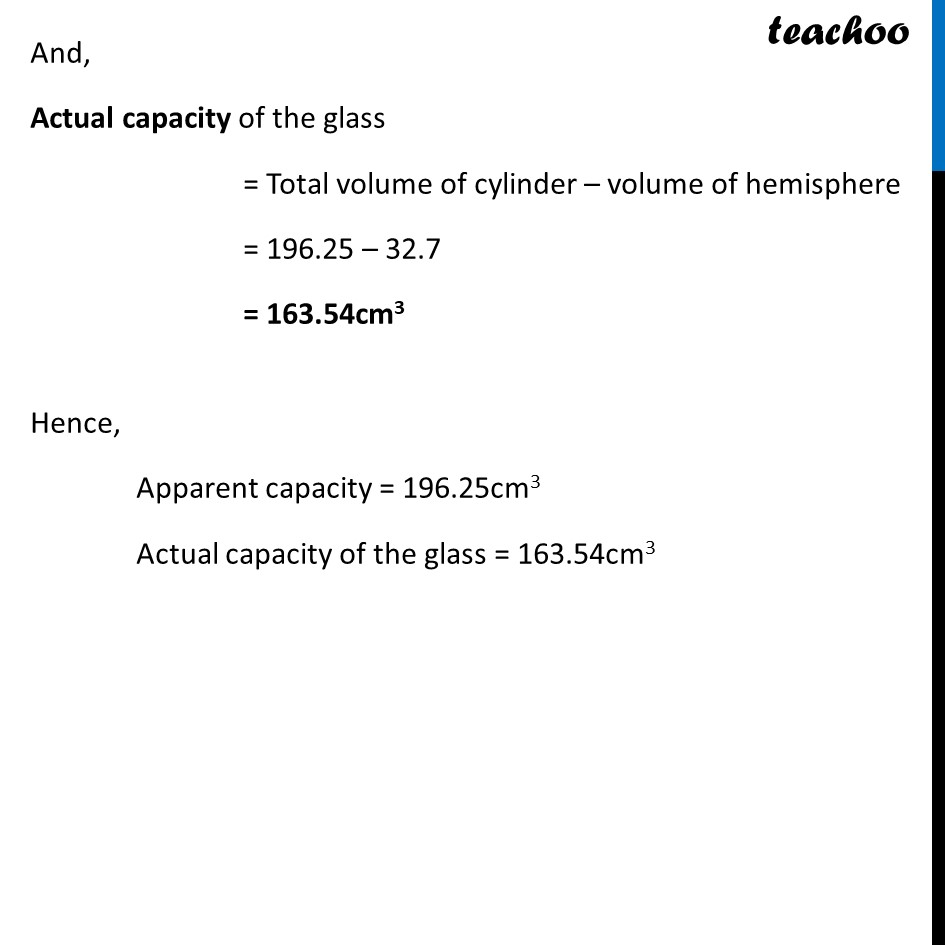Examples

Chapter 12 Class 10 Surface Areas and Volumes
Serial order wiseLearn in your speed, with individual attention - Teachoo Maths 1-on-1 Class

### Transcript

Example 6 A juice seller was serving his customers using glasses as shown in figure. The inner diameter of the cylindrical glass was 5 cm, but the bottom of the glass had a hemispherical raised portion which reduced the capacity of the glass. If the height of a glass was 10 cm, find the apparent capacity of the glass and its actual capacity. (Use π= 3.14.) Now, Apparent capacity of the glass = Volume of cylinder And, Actual capacity of the glass = Volume of cylinder – Volume of hemisphere Volume of cylinder Given inner diameter of the glass = 5 cm So, radius = r = 𝐷𝑖𝑎𝑚𝑒𝑡𝑒𝑟/2 "=" 5/2 = 2.5 cm Height = 10 cm Volume of cylinder Given Inner diameter of the glass = 5 cm So, radius = r = 𝐷𝑖𝑎𝑚𝑒𝑡𝑒𝑟/2 "=" 5/2 = 2.5 cm Height = h = 10 cm Now, Volume of the cylindrical glass = 𝜋𝑟2ℎ = 3.14 ×(2.5)2 ×10 = 3.14 × 2.5 × 2.5 ×10 = 3.14 × 6.25 × 10 = 196.25 cm3 Volume of the hemisphere Radius of hemisphere = r = 2.5 cm Volume of the hemisphere = 2/3 πr3 = 𝟐/𝟑 × 𝟑.𝟏𝟒 × (𝟐.𝟓)𝟑 = 2/3×3.14×2.5×2.5×2.5 = 2/3×3.14×15.62 = 32.7 cm3 Now, Apparent capacity of glass = Volume of cylinder = 196.25 cm3 And, Actual capacity of the glass = Total volume of cylinder – volume of hemisphere = 196.25 – 32.7 = 163.54cm3 Hence, Apparent capacity = 196.25cm3 Actual capacity of the glass = 163.54cm3